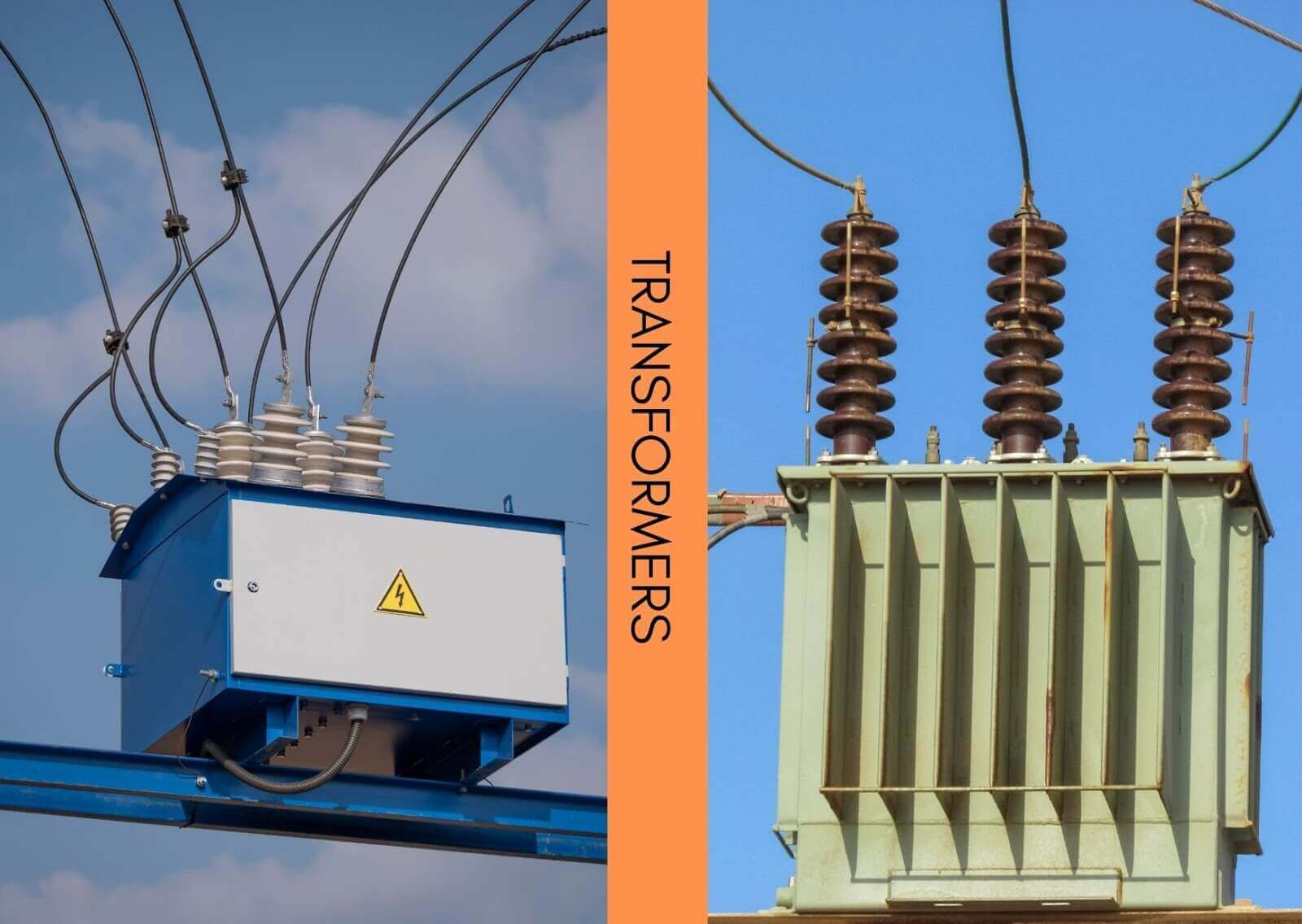# Transformer Calculator

Select the phase, choose the term you want to calculate, enter values of required input fields, and hit calculate button using transformer calculator## Transformer calculator

Input the values of ampere and voltage. Then to calculate KVA (rating) choose between three-phase and single-phase from the Transformer calculator.

This 3 in 1 calculator helps users to find the current, voltage, and rated phase power of the step up and step down transformer. you can choose to find any of these values.

## What is a transformer?

A passive electrical device that transmits a power supply. A transformer basically transfers electricity from one circuit to multiple or other circuits.• Step-up transformers have higher secondary voltage than the primary voltage.
• Step-down transforms have higher primary voltage.

## Transformer Rating

Transformer rating describes the capability of the transformer to handle voltage and current. It is necessary to know to save any damage to the powerline.

Transformer rating is calculated in kVA (KiloVolt Amperes). During the transformer sizing, the calculator above can come in handy to compute kVA.

## Transformer Rating Formula

The formula of kVA for a single-phase is:

kVA = (V x I) / 1000

The formula for a three-phase transformer is:

kVA = (3.V x I) / 1000

In these equations:

• kVA is the power rating.
• V is voltage.
• I is current.

## How to calculate kVA?

Example:

Calculate kVA of a three-phase transformer if:

V = 200

A = 130

Solution:

kVA = (√3. V x I ) / 1000

kVA = (√3. 200 x 130 ) / 1000

kVA = 45,033/1000

kVA = 45